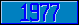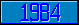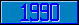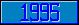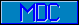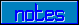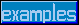## Equals sign ("=")

ASCII code 61.

This character may be used as an 'assignment' operator.
Set X="Look here!"

This character may be used as a binary 'is equal to' operator.
If X=5

This character may be used as part of a binary 'is not equal to' operator.
If X'=5

Approved for addition in a future M[UMPS] language standard:

This character may be used as part of a binary 'is less than or equal to' operator.
If X<=5

This character may be used as part of a binary 'is not less than or equal to' operator.
If X'<=5

This character may be used as part of a binary 'is greater than or equal to' operator.
If X>=5

This character may be used as part of a binary 'is not greater than or equal to' operator.
If X'>=5